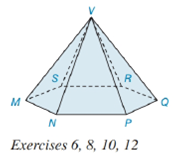Chapter 9.2, Problem 6EElementary Geometry For College St...

7th Edition
Alexander + 2 others
ISBN: 9781337614085

Solutions

Chapter
SectionElementary Geometry For College St...

7th Edition
Alexander + 2 others
ISBN: 9781337614085
Textbook Problem

In the pyramid shown in Exercise 6 on page 416, the base is a regular hexagon.a) Name the vertex (apex) of the pyramid.b) Name the base edges of the pyramid.c) Assuming the lateral edges are congruent, are the lateral faces also congruent?d) Assuming the lateral edges are congruent, is the solid a regular hexagonal pyramid?To determine

(a)

To name:

The vertex in the given figure.

Explanation

Given:

The figure given below

and base is a regular hexagon.

Properties Used:

A pyramid is made by connecting a base to an apex or vertex. The base is flat with straight edges, no curves, hence, a polygon and all other faces are triangles. The altitude/height of the pyramid is the line segment from the vertex perpendicular to the plane of the base.

To determine

(b)

To name:

The base edges in the given figure.

To determine

(c)

To find:

Whether the lateral faces are congruent in the given figure.

To determine

(d)

To find:

Whether the given figure is a regular hexagonal pyramid.

Still sussing out bartleby?

Check out a sample textbook solution.

See a sample solution

The Solution to Your Study Problems

Bartleby provides explanations to thousands of textbook problems written by our experts, many with advanced degrees!

Get Started

Find the distance between (2, 4) and (6, 8).

Applied Calculus for the Managerial, Life, and Social Sciences: A Brief Approach

Evaluate the integral. 18. ecos2d

Calculus: Early Transcendentals

Evaluate the integral. 35. 12x4(lnx)2dx

Single Variable Calculus: Early Transcendentals